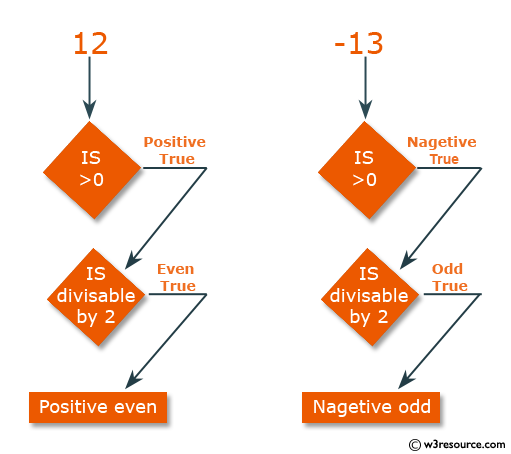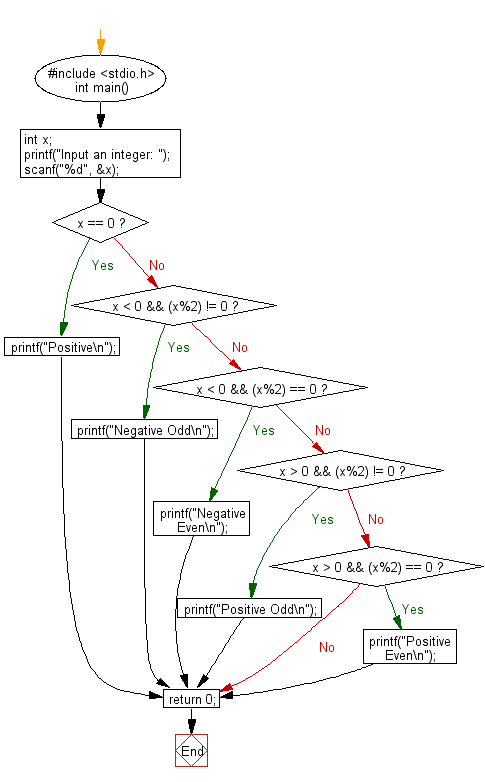﻿ C : Check an integer is positive even-odd, negative even-odd

# C Exercises: Check whether a given integer is positive even, negative even, positive odd or negative odd

## C Basic Declarations and Expressions: Exercise-31 with Solution

Write a C program to check whether a given integer is positive even, negative even, positive odd or negative odd. Print even if the number is 0.

Pictorial Prresentation:C Code:

``````#include <stdio.h>
int main() {
int x; // Declare a variable to store user input
printf("Input an integer: ");
scanf("%d", &x); // Prompt user for an integer

// Check different conditions based on the value of x
if(x == 0){
printf("Positive\n");
}
else if(x < 0 && (x%2) != 0) // Check if x is negative and odd
{
printf("Negative Odd\n");
}
else if(x < 0 && (x%2) == 0) // Check if x is negative and even
{
printf("Negative Even\n");
}
else if(x > 0 && (x%2) != 0) // Check if x is positive and odd
{
printf("Positive Odd\n");
}
else if(x > 0 && (x%2) == 0) // Check if x is positive and even
{
printf("Positive Even\n");
}

return 0;
}
``````

Sample Output:

```Input an integer: 13
Positive Odd
```

Flowchart:C Programming Code Editor:

What is the difficulty level of this exercise?

Test your Programming skills with w3resource's quiz.

﻿